# New Features in CUDA 7.5Today I’m happy to announce that the CUDA Toolkit 7.5 Release Candidate is now available. The CUDA Toolkit 7.5 adds support for FP16 storage for up to 2x larger data sets and reduced memory bandwidth, cuSPARSE GEMVI routines, instruction-level profiling and more. Read on for full details.

## 16-bit Floating Point (FP16) Data

CUDA 7.5 expands support for 16-bit floating point (FP16) data storage and arithmetic, adding new half and half2 datatypes and intrinsic functions for operating on them. 16-bit “half-precision” floating point types are useful in applications that can process larger datasets or gain performance by choosing to store and operate on lower-precision data. Some large neural network models, for example, may be constrained by available GPU memory; and some signal processing kernels (such as FFTs) are bound by memory bandwidth.

Many applications can benefit by storing data in half precision, and processing it in 32-bit (single) precision. At GTC 2015 in March, NVIDIA CEO Jen-Hsun Huang announced that future Pascal architecture GPUs will include full support for such “mixed precision” computation, with FP16 (half) computation at higher throughput than FP32 (single) or FP64 (double) .

With CUDA 7.5, applications can benefit by storing up to 2x larger models in GPU memory. Applications that are bottlenecked by memory bandwidth may get up to 2x speedup. And applications on Tegra X1 GPUs bottlenecked by FP32 computation may benefit from 2x faster computation on half2 data.

CUDA 7.5 provides 3 main FP16 features:

1. A new header, cuda_fp16.h defines the half and half2 datatypes and __half2float() and __float2half() functions for conversion to and from FP32 types, respectively.
2. A new cublasSgemmEx()“ routine performs mixed-precision matrix-matrix multiplications using FP16 data (among other formats) as inputs, while still executing all computation in full 32-bit precision. This allows multiplication of 2x larger matrices on the GPU.
3. For current users of Drive PX with Tegra X1 GPUs (and on future GPUs such as Pascal), cuda_fp16.h also defines intrinsics for 16-bit computation and comparison. cuBLAS also includes cublasHgemm() (half-precision computation matrix-matrix multiply) routine for these GPUs.

NVIDIA GPUs implement the IEEE 754 floating point standard (2008), which defines half-precision numbers as follows (see Figure 1).

• Sign: 1 bit
• Exponent width: 5 bits
• Significand precision: 11 bits (10 explicitly stored)

The range of half-precision numbers is approximately$5.96 \times 10^{-8} \ldots 6.55 \times 10^4$. half2 structures store two half values in the space of a single 32-bit word, as the bottom of Figure 1 shows.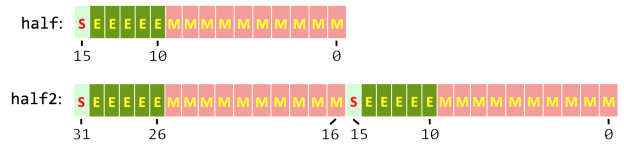Figure 1: 16-bit half-precision data formats. Top: single half value. Bottom: half2 vector representation.

## New cuSPARSE Routines Accelerate Natural Language Processing.

The cuSPARSE library now supports the cusparse{S,D,C,Z}gemvi() routine, which multiplies a dense matrix by a sparse vector, using the following equation.$\mathbf{y} = \alpha op(\mathbf{A}) \mathbf{x} + \beta \mathbf{y},$

where$\mathbf{A}$ is a dense matrix,$\mathbf{x}$ is a sparse input vector,$\mathbf{y}$ is a dense output vector, and op() is either a no-op, transpose, or conjugate transpose. For example:$\left[ \begin{array}{c} \mathbf{y}_1 \\ \mathbf{y}_2 \\ \mathbf{y}_3 \end{array} \right] = \alpha \left[ \begin{array}{ccccc} \mathbf{A}_{11} & \mathbf{A}_{12} & \mathbf{A}_{13} & \mathbf{A}_{14} & \mathbf{A}_{15} \\ \mathbf{A}_{21} & \mathbf{A}_{22} & \mathbf{A}_{23} & \mathbf{A}_{24} & \mathbf{A}_{25} \\ \mathbf{A}_{31} & \mathbf{A}_{32} & \mathbf{A}_{33} & \mathbf{A}_{34} & \mathbf{A}_{35} \end{array} \right] \left[ \begin{array}{c} - \\ 2 \\ - \\ - \\ 1 \end{array} \right] + \beta \left[ \begin{array}{c} \mathbf{y}_1 \\ \mathbf{y}_2 \\ \mathbf{y}_3 \end{array} \right]$

This type of computation is useful in machine learning and natural language processing applications. Suppose I’m processing English language documents, so I start with a dictionary, which assigns a unique index to every word in the English language. If the dictionary has$N$ entries, then any document can be represented with a Bag of Words (BoW): an$N$-dimensional vector in which each entry is the number of occurences of the corresponding dictionary word in the document.

In natural language processing and machine translation, it’s useful to compute a vector representation of words, where the vectors have O(300) dimensions (rather than a raw BoW representation which may have hundreds of thousands of dimensions, due to the size of the language dictionary). A good example of this approach is the word2vec algorithm, which maps natural language words into a semantically meaningful vector space. In word2vec, similar words map to similar locations in the vector space, which aids reasoning about word relationships, pattern recognition, and model generation.

Mapping a sentence or document represented as a BoW into the lower-dimensional word vector space requires multiplying a dense matrix with a sparse vector, where each row in the matrix corresponds to the vector corresponding to a dictionary word, and the vector is the sparse BoW vector for the sentence/document.

The new cusparse{S,D,C,Z}gemvi() routine in CUDA 7.5 makes it easier for developers of these complex applications to achieve high performance with GPUs. cuSPARSE routines are tuned for top performance on NVIDIA GPUs, so users don’t need to be experts in GPU performance.

To learn more about related techniques in machine translation, check out the recent post Introduction to Neural Machine Translation.

## Pinpoint Performance Bottlenecks with Instruction-Level Profiling

One of the biggest challenges in optimizing code is determining where in the application to put optimization effort for the greatest impact. NVIDIA has been improving profiling tools with every release of CUDA, adding more focused introspection and smarter guided analysis. CUDA 7.5 further improves the power of the NVIDIA Visual Profiler (and NSight Eclipse Edition) by enabling true instruction-level profiling on Maxwell GM200 and later GPUs. This lets you quickly identify the specific lines of source code causing performance bottlenecks in GPU code, making it easier to apply advanced performance optimizations.

Before CUDA 7.5, the NVIDIA Visual Profiler supported kernel-level profiling: for each kernel, the profiler could tell you the amount of time spent, the relative importance as a fraction of total run time, and key statistics and limiters. For example, Figure 1 shows a kernel-level analysis showing that the kernel in question is possibly limited by instruction latencies.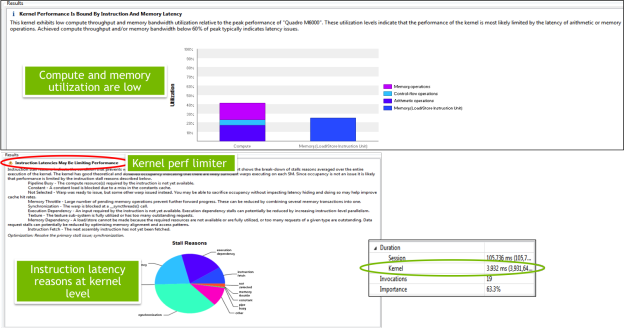Figure 2: Before CUDA 7.5, the NVIDIA Visual Profiler supported only kernel-level profiling, showing performance and key statistics and limiters for each kernel invocation. Click for full resolution.

CUDA 6 added support for more detailed profiling, correlating lines of code with the number of instructions executed by those lines, as Figure 2 shows. But the highest instructions count lines do not necessarily take the longest. In the example, these lines from a reduction are not taking as long as the true hotspot, which has longer stalls due to memory dependencies.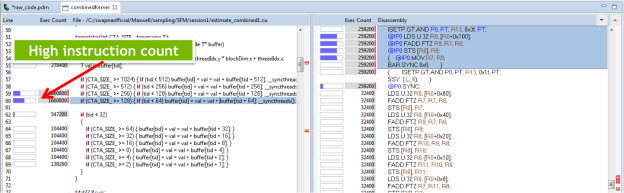Figure 3: CUDA 6 added support for detailed profiling, showing the correspondence between source lines and assembly code, and the number of instructions executed for each source line. Click for full resolution.

Per-kernel statistics and instruction counts are very useful information, but getting to the root of performance problems in complex kernels could still be difficult. When profiling, you want to know exactly which lines are taking the most execution time. With CUDA 7.5, the profiler uses program counter sampling to find and show specific “hot spot” lines of code where the kernel is spending most of its time, as Figure 3 shows.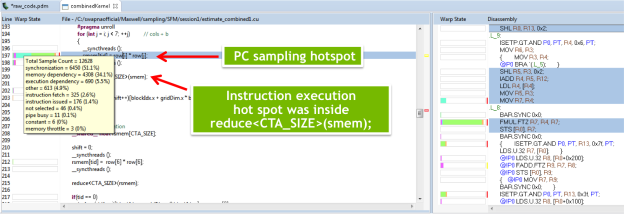Figure 3: New in CUDA 7.5, instruction-level-profiling pinpoints specific lines of code that are hotspots. Click for full resolution.

Not only does the profiler show hotspot lines, but it shows potential reasons for the hotspot, based on the state of warps executing the lines. In this case, the hotspot is due to synchronization and memory latency, and the assembly view shows that the kernel is stalling on local memory loads (LDL) and __syncthreads(). Knowing this, the kernel developer can optimize the kernel to keep data in registers. Figure 4 shows the results after code tuning, where the kernel time has improved by about 2.5x.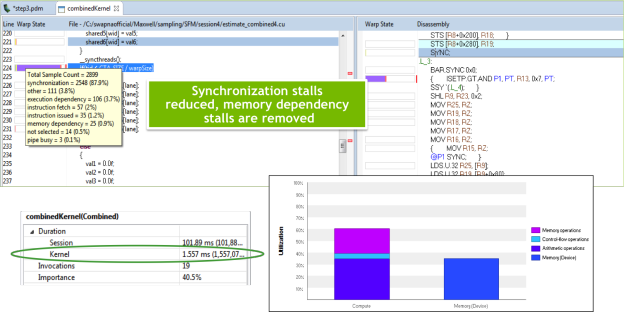Figure 4: By using instruction-level profiling, the developer was able to optimize the kernel performance, achieving a 2.5X kernel speedup. Click for full resolution.

## Experimental Feature: GPU Lambdas

CUDA 7 introduced support for C++11, the latest version of the C++ language standard. Lambda expressions are one of the most important new features in C++11. Lambda expressions provide concise syntax for defining anonymous functions (and closures) that can be defined in line with their use, can be passed as arguments, and can capture variables.

C++11 lambdas are handy when you have a simple computation that you want to use as an operator in a generic algorithm, like the thrust::count_if() algorithm that I used in a past blog post. The following code from that post uses Thrust to count the frequency of ‘x’, ‘y’, ‘z’, and ‘w’ characters in a text. But before CUDA 7.5, this could only be done with host-side lambdas, meaning this code couldn’t execute on the GPU.

#include <initializer_list>

void xyzw_frequency_thrust_host(int *count, char *text, int n)
{
using namespace thrust;

*count = count_if(host, text, text+n, [](char c) {
for (const auto x : { 'x','y','z','w' })
if (c == x) return true;
return false;
});
}


CUDA 7.5 introduces an experimental feature: GPU lambdas. GPU lambdas are anonymous device function objects that you can define in host code, by annotating them with a __device__ specifier. Here is xyzw_frequency function modified to run on the GPU. The code indicates the GPU lambda with the __device__ specifier before the parameter list.

#include <initializer_list>

void xyzw_frequency_thrust_device(int *count, char *text, int n)
{
using namespace thrust;

*count = count_if(device, text, text+n, [] __device__ (char c) {
for (const auto x : { 'x','y','z','w' })
if (c == x) return true;
return false;
});
}


### Parallel For Programming

GPU lambdas enable a “parallel-for” style of programming that lets you write parallel computations in-line with the code that invokes them—just like you would with a for loop. The following SAXPY shows how for_each() lets you write parallel code for a GPU in a style very similar to a simple for loop. Using Thrust in this way ensures you get great performance on the GPU, as well as performance portability to CPUs: the same code can be compiled and run for multi-threaded execution on CPUs using Thrust’s OpenMP or TBB backends.

void saxpy(float *x, float *y, float a, int N) {
using namespace thrust;
auto r = counting_iterator(0);
for_each(device, r, r+N, [=] __device__ (int i) {
y[i] = a * x[i] + y[i];
});
}


GPU lambdas are an experimental feature in CUDA 7.5. To use them, you need to enable the feature by passing the flag --expt-extended-lambda to nvcc on the compiler command line. As an experimental feature, GPU lambda functionality is subject to change in future releases, and there are some limitations to how they can be used. See the CUDA C++ Programming Guide for full details. I’ll write more about GPU lambdas in a future blog post.

## Windows Remote Desktop

With CUDA 7.5, you can now run Windows CUDA applications remotely via Windows Remote Desktop. This means that even without a CUDA-capable GPU in your Windows laptop, you can still run GPU-accelerated applications remotely on a Windows server or desktop PC. CUDA applications can also now be run as services on Windows.

These Windows capabilities are supported on all NVIDIA GPU products.

## LOP3

A new LOP3 instruction is added to PTX assembly, supporting a range of 3-operand logic operations, such as A & B & C, A & B & ~C, A & B | C, etc. This functionality, supported on Compute Capability 5.0 and higher GPUs, can save instructions when performing complex logic operations on multiple inputs. See section 8.7.7.6 of the PTX ISA specification included with the CUDA Toolkit version 7.5.

## More improvements

• 64-bit API for cuFFT
•$n$-dimensional Euclidian norm floating-point math functions
• Bayer CFA to RGB conversion functions in NPP
• Faster double-precision square-roots (sqrt)
• Programming examples for the cuSOLVER library
• Nsight Eclipse Edition supports the POWER platform

## Platform Support

The CUDA 7.5 release notes include a full list of supported platforms; here are some notable changes.

• Added: Ubuntu 15.04, Windows 10, and (upcoming) OS X 10.11
• Added: host compiler support for Clang 3.5 and 3.6 on Linux.
• Removed: Ubuntu 12.04 LTS on (32-bit) x86, cuda-gdb native debugging on Mac OS X
• Deprecated: legacy (environment variable-based) command-line profiler. Use the more capable nvprof` command-line profiler instead.